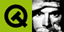Home | All Classes | Main Classes | Annotated | Grouped Classes | Functions# TQRegExpValidator Class Reference

The TQRegExpValidator class is used to check a string against a regular expression. More...

#include <ntqvalidator.h>

Inherits TQValidator.

## Detailed Description

The TQRegExpValidator class is used to check a string against a regular expression.

TQRegExpValidator contains a regular expression, "regexp", used to determine whether an input string is Acceptable, Intermediate or Invalid.

The regexp is treated as if it begins with the start of string assertion, ^, and ends with the end of string assertion \$ so the match is against the entire input string, or from the given position if a start position greater than zero is given.

For a brief introduction to TQt's regexp engine see TQRegExp.

Example of use:

```    // regexp: optional '-' followed by between 1 and 3 digits
TQRegExp rx( "-?\\d{1,3}" );
TQValidator* validator = new TQRegExpValidator( rx, this );

TQLineEdit* edit = new TQLineEdit( this );
edit->setValidator( validator );
```

Below we present some examples of validators. In practice they would normally be associated with a widget as in the example above.

```    // integers 1 to 9999
TQRegExp rx( "[1-9]\\d{0,3}" );
// the validator treats the regexp as "^[1-9]\\d{0,3}\$"
TQRegExpValidator v( rx, 0 );
TQString s;
int pos = 0;

s = "0";     v.validate( s, pos );    // returns Invalid
s = "12345"; v.validate( s, pos );    // returns Invalid
s = "1";     v.validate( s, pos );    // returns Acceptable

rx.setPattern( "\\S+" );            // one or more non-whitespace characters
v.setRegExp( rx );
s = "myfile.txt";  v.validate( s, pos ); // Returns Acceptable
s = "my file.txt"; v.validate( s, pos ); // Returns Invalid

// A, B or C followed by exactly five digits followed by W, X, Y or Z
rx.setPattern( "[A-C]\\d{5}[W-Z]" );
v.setRegExp( rx );
s = "a12345Z"; v.validate( s, pos );        // Returns Invalid
s = "A12345Z"; v.validate( s, pos );        // Returns Acceptable
s = "B12";     v.validate( s, pos );        // Returns Intermediate

rx.setCaseSensitive( FALSE );
v.setRegExp( rx );
s = "readme";      v.validate( s, pos ); // Returns Acceptable
s = "README.1ST";  v.validate( s, pos ); // Returns Acceptable
s = "read me.txt"; v.validate( s, pos ); // Returns Invalid
s = "readm";       v.validate( s, pos ); // Returns Intermediate
```

## Member Function Documentation

### TQRegExpValidator::TQRegExpValidator ( TQObject * parent, const char * name = 0 )

Constructs a validator that accepts any string (including an empty one) as valid. The object's parent is parent and its name is name.

### TQRegExpValidator::TQRegExpValidator ( const TQRegExp & rx, TQObject * parent, const char * name = 0 )

Constructs a validator which accepts all strings that match the regular expression rx. The object's parent is parent and its name is name.

The match is made against the entire string, e.g. if the regexp is [A-Fa-f0-9]+ it will be treated as ^[A-Fa-f0-9]+\$.

### TQRegExpValidator::~TQRegExpValidator ()

Destroys the validator, freeing any resources allocated.

### const TQRegExp & TQRegExpValidator::regExp () const

Returns the regular expression used for validation.

### void TQRegExpValidator::setRegExp ( const TQRegExp & rx )

Sets the regular expression used for validation to rx.

### TQValidator::StateTQRegExpValidator::validate ( TQString & input, int & pos ) const [virtual]

Returns Acceptable if input is matched by the regular expression for this validator, Intermediate if it has matched partially (i.e. could be a valid match if additional valid characters are added), and Invalid if input is not matched.

The pos parameter is set to the length of the input parameter.

For example, if the regular expression is \w\d\d (that is, word-character, digit, digit) then "A57" is Acceptable, "E5" is Intermediate and "+9" is Invalid.